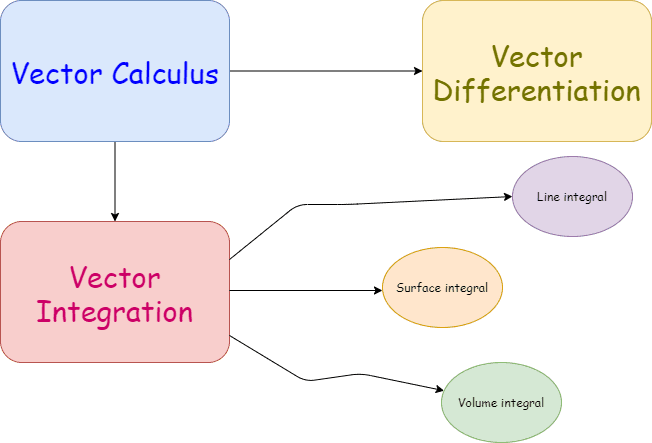Categories
College Mathematics

# Line Integral : IIT JAM 2018 Question Number 20

Try this beautiful problem from IIT JAM 2018 . It involves calculating the line integral of a vector point function along a closed curve. We provide sequential hints so that you can try this problem by yourself

# What are we learning ?

Competency in Focus: Application of Calculus This is problem from IIT JAM 2018 is based on calculation of Line integration of a vector point function along a closed curve.

# First look at the knowledge graph.# Next understand the problem

If  $\vec{F}(x,y)=(3x-8y)\widehat{i}+(4y-6xy)\widehat{j}$ for $(x,y) \in \mathbb{R}^2$, then $\oint \vec{F}. \mathrm d \vec{r}$, where $C$ is the boundary of triangular region bounded by the lines $x=0,y=0,\quad \textbf{and} \quad x+y=1$ oriented in the anti clock wise direction is    $(A)\quad \frac{5}{2} \qquad (B)\quad 3 \qquad (C)\quad 4 \qquad (D)\quad 5 \qquad$
##### Source of the problem
IIT JAM 2018, Question number 20
##### Key Competency
Calculation of line integral of a vector point function along a closed curve.
6/10
##### Suggested Book
VECTOR ANALYSIS: Schaum’s Outlines series

Do you really need a hint? Try it first!
Suppose we have vectors $\vec{u}=x_{1}\widehat{i}+y_{1}\widehat{j}+z_{1}\widehat{k}$ $\vec{v}=x_{2}\widehat{i}+y_{2}\widehat{j}+z_{2}\widehat{k}$ Then $\vec{u}.\vec{v}=x_{1}x_{2}+y_{1}y_{2}+z_{1}z_{2}$ Now in the given context $\vec{F} (x,y)=(3x-8y)\widehat{i}+(4y-6xy)\widehat{j}$ $\mathrm d \vec{r}=\mathrm d x\widehat{i}+\mathrm d y\widehat{j}$ Then $\oint_{c} \vec{F}. \mathrm d \vec{r}=\oint_c (3x-8y) \mathrm d x+(4y-6xy)\mathrm d y$ Now we have to chose the proper path to complete the line integral. Do you want to try from here?
The region is bounded by $x=0=y$ & $x+y=1$ i.e.,Let us divide the segment into 3 parts where $I_1 : y=0 ; \quad I_2 : x+y=1; \quad I_3 : x=0$ i.e., $\oint_c \vec{F}. \mathrm d \vec{r} = \oint_{I_1} \vec{F}. \mathrm d \vec{r} + \oint_{I_2} \vec{F}. \mathrm d \vec{r}+\oint_{I_3} \vec{F}. \mathrm d \vec{r}$   Let us calculate $\oint_{I_1} \vec{F}. \mathrm d \vec{r}$ & $\oint_{I_3} \vec{F}. \mathrm d \vec{r}$ and I’ll keep $\oint_{I_2} \vec{F}. \mathrm d \vec{r}$  for your try by the end of this hint. $\oint_{I_1} \vec{F}. \mathrm d \vec{r}=\int_0^1 3x \mathrm d x$ [We have put $y=0$ so $\mathrm d y=0$ , Hence the limit will be on $x$] $=\frac{3}{2}$ $\oint_{I_3} \vec{F}. \mathrm d \vec{r}=\int_1^0 4y \mathrm d y$ [We have put $x=0$ so, $\mathrm d x=0$ therefore the limit will be on $y$; Observe the anticlockwise direction to understand the limit] $=[\frac{4y}{2}]_1^0$ $=-2$
On $I_2$  observe that we can transform $y$ in terms of $x$ i.e., $y=1-x$ [We can also use parameterization, which is basically the same method but I prefer this one to make the calculation faster] $y=1-x$ i.e., $\mathrm d y=-\mathrm d x$ now,  $\int_{I_2} \vec{F}.\mathrm d \vec{r}= \int_{I_2}(3x-8y)\mathrm d x+\int_{I_2}(4y-6xy)\mathrm d y$ $=\int_1^0(3x-8+8x)\mathrm d x+\int_1^0(4-4x-6x(1-x))(-\mathrm d x)$ [observe the anticlock wise direction limit] $=\int_1^0(11x-8)\mathrm d x +\int_1^0(10x-6x^2-4)\mathrm dx$ $=[\frac{11x^2}{2}-8x+\frac{10x^2}{2}-\frac{6x^3}{3}-4x]_0^1$ $=-\frac{11}{2}+8-5+2+4=\frac{7}{2}$ Hence our answer would be $\frac{3}{2}-2+\frac{7}{2}=3$

# Connected Program at Cheenta

#### Amc 8 Master class

Cheenta AMC Training Camp consists of live group and one on one classes, 24/7 doubt clearing and continuous problem solving streams.

# Similar Problems

This site uses Akismet to reduce spam. Learn how your comment data is processed.